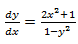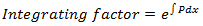Goseeko blog# What is a linear differential equation?

## Overview

An equation consisting of a differential coefficient is called a differential equation. whereas a linear equation with one or more terms, consisting of the derivatives of the dependent variable with respect to one or more independent variables is called LDE.

For example-is the differential equation.

A differential equation of the form

is known as a LDE.

The differential equation of the form dy/dx + Py = Q is the first order linear differential equation, where P and Q are the constant or function of independent variable..

P and Q, are functions of x or constants.

Few examples of LDE are-

The solution of LDE is-

Note

1.2.     If the RHS of LDE is zero for all x then it is said to be homogeneous, otherwise non-homogeneous.

## Solving a linear differential equation

1. First we re-arrange the given equation to the standard form of LDE, which is dy/dx + Py = Q is the first order linear differential equation, where P and Q are the constant or function of independent variable.

2. Then we find the integrating factor

3. Then the solution of LDE is-

Solved examples

Example: Solve

Solution-

First we will convert the given equation in standard LDE form-

Where Q = sin x and P = 2/x

Now we will find the integrating factor-

Then the solution is-

Integrating by parts-

Example: Solve-

Solution-

The given equation is already in the form of standard LDE.

Now we will find the IF-

So that the solution is-

Interested in learning about similar topics? Here are a few hand-picked blogs for you!